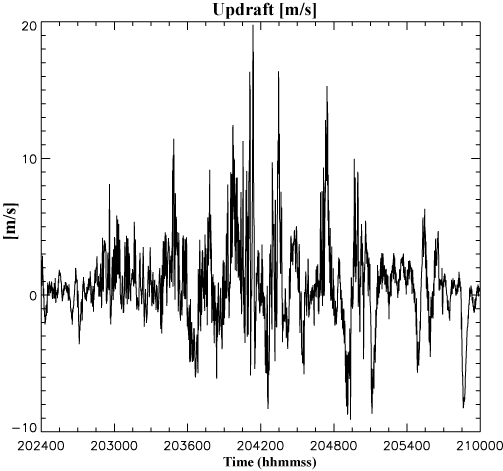#### Vertical wind

Vertical wind is approximated using a simplified inversion of the aircraft equation of motion (Kopp, 1985).  ).  An example is shown in Figure 1 for Flight 803 on June 1, 2003. The most important component of this estimate is the rate of change of aircraft pressure altitude, which is approximated as a centered difference, one second either side of the second at which the computed value is stored.

The most simplifying approximation in the equation of motion is substituting pitch (which is measured on the T-28) for angle-of-attack (which is not measured).  This approximation leads to an improvement in estimated vertical wind over that obtained using solely the rate-of-change of aircraft pressure altitude as a proxy for vertical wind, but is not as accurate as would be a similar calculation with a measured angle-of-attack. The technique is valid only for straight and level, non-accelerating flight.  The 36 min data segment below includes several turns in which the vertical wind is erroneously inferred to be negative. The positive peaks represent actual updraft penetrations. At the nominal penetration speed of 100 m s-1, each minute (each interval along the horizontal axis) represents 6 km of flight.